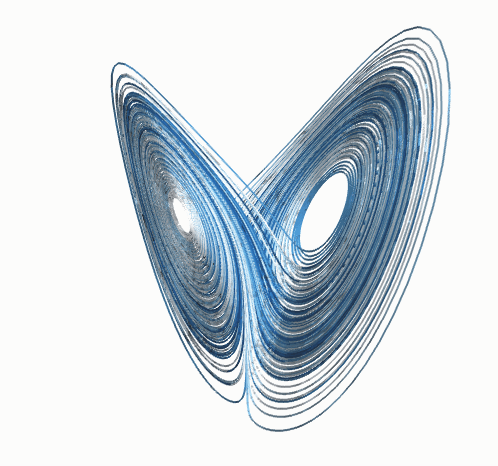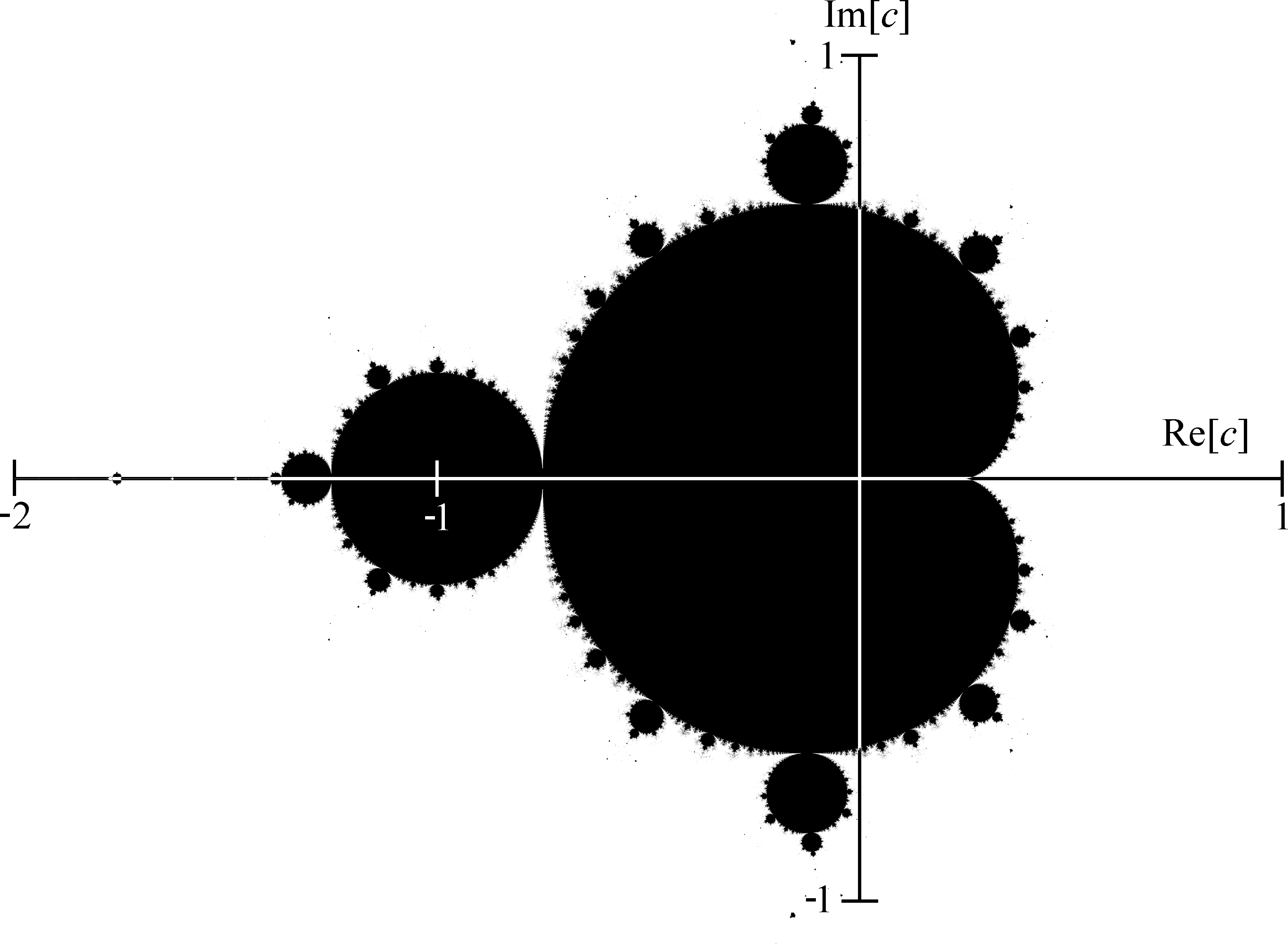# Computer science on prediction and the edge of chaos

With the development of statistical mechanics, physicists became the first agent-based modellers. Since the scientists of the 19th century didn’t have super-computers, they couldn’t succumb to the curse of computing and had to come up with analytic treatments of their “agent-based models”. These analytic treatments were often not rigorous, and only a heuristic correspondence was established between the dynamics of macro-variables and the underlying microdynamical implementation. Right before lunch on the second day of the Natural Algorithms and the Sciences workshop, Joel Lebowitz sketched how — for some models — mathematical physicists still continue their quest to rigorously show that macrodynamics fatefully reproduce the aggregate behavior of the microstates. In this way, they continue to ask the question: “when can we trust our analytic theory and when do we have to simulate the agents?”

What are the fundamental constraints on prediction? Theoretical computer scientists are probably the best equipped to answer this question in the general case. The day before Lebowitz, Cris Moore held the position of standing between the workshop participants and their lunch. He used this opportunity to explain how physics, the problem of prediction, and computational complexity relate. In computational complexity, we study what sort of problems can be solved with a limited resource such as time, space, communication, or even randomness. To start us off on the road to prediction vs. simulation, Moore considered the question of parallelization vs. serial processing ($\mathsf{NC}$ vs.$\mathsf{P}$), and finding vs. verifying an answer ($\mathsf{P}$ vs.$\mathsf{NP}$). He urged us to explore the problems in the gap between$\mathsf{NC}$ and$\mathsf{P}\mathrm{-complete}$, and$\mathsf{P}$ and$\mathsf{NP}\mathrm{-complete}$. These are the messy problems, not structured enough to encode computers, but not simple enough to fall in the lower complexity classes.In the last talk of day one, Mark Braverman considered two more obstacles to prediction: chaos and universality. Chaos theory is a well developed field of complexology that is familiar to physicists and biologists, and its limits of prediction rely on the high variance in behavior between paths with similar initial conditions. To exactly describe the behavior of a chaotic system over any significant time-horizon, the initial conditions have to be extremely well measured. In computer science terms: the initial measurement needs an exponential amount of precision in the time-horizon to which we want to predict the system. This is why we can’t predict the exact weather in a specific location to a high degree of accuracy more than a few days in the future. However, we are seldom interested in this level of precision from our models, usually we mean something else by prediction. A typical prediction might be: if we keep pumping greenhouses gasses into the atmosphere then in 100 years it will be on average far warmer on the planet. Chaotic systems do not place any fundamental constraints on such qualitative predictions, in fact they often have easy to describe long-term qualitative behavior. Universal systems pose more of a challenge.

Rice’s theorem states that for any non-trivial property (i.e. one that isn’t constant on all languages), there is no algorithm that decides if the language of a given Turning Machine satisfies that property. For natural algorithms, this means that if your model is universal (there is a way to use the initial conditions and parameters of your model to encode an arbitrary Turing Machine) then, in general, even qualitative statements about the long-term behavior of your system are impossible. If you are a modeler, and you built a model that is universal then you’ve simply built in too much freedom, you can recreate anything and predict nothing. As with Moore, there are problems in the gap: undecidable models that are too unstructured to encode arbitrary Turing machines and thus not universal, but still not restricted enough to be predictable.

Universality of models is not uncommon, Bernad Chazelle‘s influence systems from the opening remarks can display both chaos and universality. In Chazelle’s model of diffusive systems, agents keep interacting with each other — contributing to the entropy of the system — and they keep trying to move towards their neighbors — contributing to the energy. If the parameters are such that entropy and energy balance perfectly then you have a criticality and features like chaos and Turing universality. However, this perfect entropy-energy balance is a knife-edge phenomena, small-disturbances tip you one way or another and the system becomes non-universal and potentially predictable. The set of parameter settings for which influence systems are unpredictable has measure zero, and a very complicated self-similar structure.

Braverman’s example had similar behavior. He considered if Julia sets — the boundary of points$z$ such that the map$z \rightarrow z^2 + c$ doesn’t grow to infinity — could be computed given a description of$c$. He found that although some Julia sets are not computable, this undecidability is not robust, applying a small noise to$c$ results in Julia sets that are computable. Like in influence systems, the undecidable Julia sets are knife-edge but a beautifully structured knife: they live on the boundary of the Mandelbrot set.In other words, if — instead of doing worst-case analysis — we look at the smoothed analysis of influence systems and Julia sets then they are predictable. It is a matter of allowing small random perturbations around the worst-case inputs: combining the best of worst-case and average-case analysis. Does this mean we can bring back statistical mechanics? The biggest intersection between statistical mechanics and traditional theoretical computer science is in looking at phase-transitions in the$\mathsf{NP}\mathrm{-complete}$ problem 3SAT. If we defined the density$\alpha$ of a 3SAT instance as the ratio of number of clauses to number of variables then as we increase$\alpha$ we transition from a state where most instances are satisfiable to one where almost none are. Near the critical values$\alpha_c$, we see the 3SAT instances that are hardest to solve, another edge of chaos. The study of this phase transition can be incredibly rewarding, but as Moore pointed out, it cannot provide us with fundamental insights into the difficulty of prediction. The 3XOR problem — a problem that easily solvable in polynomial time since it is just linear equations over$\mathbb{Z}_2$ — has the same exact statistical structure. Although statistical mechanics were the first to build analytic understanding of agent-based models, they have to pass the reins to theoretical computer scientists.Note: this is the second blogpost of a series (first here) on the recent workshop on Natural Algorithms and the Sciences at Princeton’s Center for Computational Intractability.

Chazelle, B. (2012). Natural algorithms and influence systems Communications of the ACM, 55 (12) DOI: 10.1145/2380656.2380679About Artem Kaznatcheev
From the Department of Computer Science at Oxford University and Department of Translational Hematology & Oncology Research at Cleveland Clinic, I marvel at the world through algorithmic lenses. My mind is drawn to evolutionary dynamics, theoretical computer science, mathematical oncology, computational learning theory, and philosophy of science. Previously I was at the Department of Integrated Mathematical Oncology at Moffitt Cancer Center, and the School of Computer Science and Department of Psychology at McGill University. In a past life, I worried about quantum queries at the Institute for Quantum Computing and Department of Combinatorics & Optimization at University of Waterloo and as a visitor to the Centre for Quantum Technologies at National University of Singapore. Meander with me on Google+ and Twitter.

### 12 Responses to Computer science on prediction and the edge of chaos

This site uses Akismet to reduce spam. Learn how your comment data is processed.